1.* (1997 F 2) Inorganic redox chemistry can be complicated. For example, reactions which involve the exchange of more than one electron are often very slow and sometimes do not take place, even when they are downhill from a thermodynamic point of view. This is due to the fact that electron-transfer redox precesses take place by one-electron steps. Consider the following problem: the oxidation of Tl0 to Tl3+ by Fe3+. This reaction is very slow. Use the standard redox potentials given below to evaluate the proposed reaction:

3Fe3+ + Tl0 ---> 3Fe2++ Tl3+

Eo values:

Tl3+/Tl1+ E0 = +1.25V
Tl1+/Tl0 E0 = -0.336V
Tl3+/Tl0 E0 = +0.72V
Fe3+/Fe2+ E0 = +0.77V

A. Evaluate the thermodynamic feasibility of the proposed reaction.

B. Provide a rational for the fact that the proposed reaction is too slow to observe.

2.* (1997 F 11) Adenine triphosphate (ATP) is the principal immediate donor of free energy in biological reactions. In a cell, ATP is being formed and consumed all the time; the typical delay between formation and consumption is approximately one minute. The turnover rate of ATP is remarkably high. A resting human consumes about 40kg of ATP in 24 hours, which is an appreciable fraction of the total body weight! A large amount of free energy is liberated when ATP is hydrolyzed to adenine diphosphate (ADP) and orthophosphate (Pi).

ATP + H2O ---> ADP + H+ +Pi   DG0 = -16.8 kcal/mol

To understand the role of ATP in free energy coupling, consider the reaction

X ---> Y    DG0 = +7 kcal/mol

A. Calculate the equilibrium constant K = [Y]/[X] for this reaction. Use R = 2cal/Kmol.

B. Thus X cannot be spontaneously converted to Y when the molar ratio of [Y]/[X] is equal to or greater than K. But X can be converted to Y when the [Y]/[X] ratio is coupled to the hydrolysis to ATP. The new overall reaction is

X + ATP + H2O --> Y + ADP + H+ +Pi   DG = -9.8 kcal/mol

Where the value of DG0 for this reaction is the sum of the DG0 values for the two separate reactions. Under the conditions of a coupled reaction K’ = ([Y]/[X])([ADP][Pi][H+]/[ATP]). Find the value of K’.

C. The ATP-generating system of cells maintains the [ATP]/([ADP][Pi]H+] ratio at a high level, typically on the order of 500. Use this information to calculate [Y]/[X] for the coupled reaction.

D. By comparing your results for parts A and C, determine by how much the hydrolysis of an ATP molecule changes the equilibrium ratio of [Y]/[X] when it is a coupled reaction compared to an uncoupled reaction.

E. Here is a system analogous to metabolic thermodynamics. Suppose compound A is broken down into E by the following three-step reaction sequence:

 DG0 (kcal/mol) A + B --->> C -3 C ---> D -55 D ---> B + E -32

What is the standard free energy change for the reaction A ---> E?

F. Coupled to the second step is the reaction F -> G for which DG0 = +60 kcal/mol. Explain how it is possible that a reaction with DG0 of 60 kcal/mol can be made to go by one of only —55 kcal/mol.

G. Assuming equimolar concentrations of F and G, what must be the relative concentration of C and D for perfect free energy coupling between C ---> D and F ---> G reactions, that is, so that the overall DG0 value is zero?

H. Demonstrate rigorously that the total free energy change in one turn of the cycle is independent of the concentrations of B, C, and D.

3.* (1997 F 14) The following battery (galvanic cell) is constructed.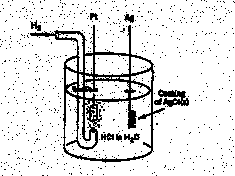This battery may be regarded as the combination of two half cells:

H+ (aq) + e- = 1/2 H2(g) E0 = 0.00V

AgCl(s) + e- = Ag(s) + Cl- (aq) E0 = 0.22V

Where the concentrations for H+(aq) and Cl-(aq) are 1M, the H2 (g) pressure is 1 atm, and the temperature is 250C.

Actually, an extremely careful study has been made of this battery reaction by R. G. Bates and V. E. Bower, who measured the standard electromotive force E0 in volts as a function of temperature t in degrees centigrade. Their data may be represented as follows.

E0/V = 0.23659-4.8564 x 10-4 (t/0C) — 3.4205 x 10-6 (t/0C) 2 + 5.869 x 10-9 (t/0C) 3

A. Use this data to find DG0 for the reaction 1/2 H2(g) + AgCl(s) --> H+ (aq) + Cl- (aq) + Ag(s) recalling that the Faraday constant is 96485 coulombs/mole, the universal gas constant R=8.314J/Kmol, and 1 coulomb-volt = 1Joule.

B. Write down an expression for the eqilibrium constant K at 25oC for this reaction and find the value of K.

C. Use the data provided to find DS0 for this battery reaction.

D. Under standard state conditions is this battery reaction driven by entropy change, driven by enthalpy change, or driven by both entropy and enthalpy changes? Explain.

4.* (1997 3 3) An important class of enzymes, the cytochrome P450 family, catalyzes the reaction between molecular oxygen and hydrocarbons producing alcohols. It may seem surprising that these enzymes use a 2e- reducing agent to activate oxygen such that the overall stoichiometry is as follows: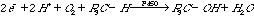In fact, it is often found that oxidation reactions using oxygen also involve a 2e- reducing agent. You may find the following standard reduction poentials for oxygen (given in volts under standard conditions: 250C, [H+]=1M, [H2]=1 atm) to be useful.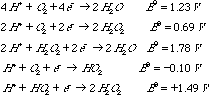A. Explain how a reducing agent can make oxygen into a more powerful oxidant. Use thermodynamic arguments.

B. The protonated form of superoxide anion, HO2-, is unstable; it disproportionates for form hydrogen peroxide and oxygen. Show that this reaction is thermodynamically downhill under standard conditions.

C. Three oxidation states of molecular oxygen (O2) are shown in the table of reduction potentials. Some of these are paramagnetic. Place an asterisk on those paramagnetic species.

5.* (1996 F 9) Consider the gases N2O, O2, and O3. Half-cell potentials are given for reduction of these substances in acid medium.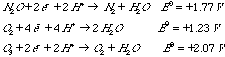A. Arrange the substances in order of decreasing oxidizing power.

B. N2O ("laughing gas") is used as an anesthetic. It is rather inert and poses no danger of explosion in the operating theatre. For example, N2O does not react with H2. Is this what you expect based on the above potentials? Explain.

6.* (1996 F 10E) Name the most powerful oxidizing agent in chemistry.

7.* (1996 F 11B) Write a balanced equation for the decomposition of ozone. What is the sign of DG, DH, and DS?

8.* (1996 F 15) The dimerization of nitrogen dioxide results in measurable amounts of reactant and product molecules at 250C. The reaction is: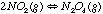A. Write an expression for the equilibrium constant K.

B. The value of K at 250C is 8.8. A mixture of the two gases is sampled and the partial pressures are found to be 9.8 x 10-2 atm for NO2 (g) and 2.1 x 10-1 atm for N2O4(g). Is this system at equilibrium? If not, what will be the spontaneous direction of change?

C. What is the sign of DH°?

D. What is the sign of DS°?

E. Predict how the equilibrium constant will change with temperature.

9.* (1996 3 2) Consider the thermodynamic factors underlying the polymerization of a simple olefin: propylene.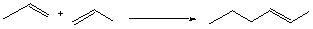A. Calculate the value of the enthalpy change DH° (the bond energies of a carbon-carbon sigma bond and a carbon-carbon p bond are approximately 86 and 63 kcal/mol, respectively).

B. Give the sign of the enthalpy change for the polymerization.

C. Deduce the sign of the entropy change for this reaction.

D. What is the sign of the Gibbs free energy change for this reaction? Explain your reasoning and show your calculation.

10.* (1996 3 4) Solubility depends on temperature. When solid potassium nitrate KNO3(s) is dissolved in water the process reaches equilibrium

heat + KNO3 (s) + H2O(l) < -- > K+(aq) + NO3-(aq) (1)

This dissolving process occurs with the absorption of head (endothermic process). When gaseous O2 is dissolved in water, the process reaches equilibrium

O2 (gas) + H2O(l) < -- > O2 (aq) + heat (2)

A. For reaction 1 what is the sign of DH?

B. For reaction 2 what is the sign of DH?

C. If a saturated solution of potassium nitrate is in contact with solid KNO3 and the solution is heated, will the solubility of KNO3 increase, decrease, or stay the same?

D. If a saturated solution of oxygenated water is in contact with gaseous oxygen and the solution is heated, will the solubility of O2 increase, decrease, or stay the same?

E. Explain in thermodynamic terms why KNO3 (s) and O2 (g) both dissolve spontaneously in pure water.

11.* (1996 3 6) You are given the following reactions with their standard potentials as shown.

 Reactions Voltage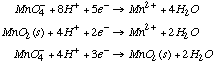E°1=+1.57V E°2=+1.23V E°3=????V (1) (2) (3)

A. Find E°3.

B. What is E°4 for the same reaction as (3) but run under basic conditions rather than acidic conditions? The new half reaction is: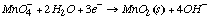E°4=????V (4)

12.* (1995 F 2G) Give two examples of oxidizing agents more powerful than molecular oxygen.

13.* (1995 F 3) For the following pairs, which has the higher entropy or more positive entropy change?

A.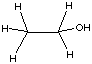a.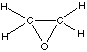b.

B.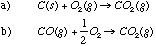C.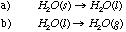14.* (1995 F 5)

A. What are the conditions on the sign of DH and DS so that:

1. the reaction is always spontaneous?
2. the reaction occurs spontaneously at low temperature but not at high temperature?
3. the reaction occurs spontaneously at high temperature but not at low temperature?
4. the reaction never occurs spontaneously?
5. the reaction is reversible

B. If a reaction is nonspontaneous, how would addition of a catalyst affect yield?

15.* (1995 F 8) The following are the four bases found in DNA.A. Draw the complementary base pairs indicating their hydrogen bonding.

B. The double-stranded helical structure of DNA is maintained primarily by the hydrogen bonds, which are weak bonds. With increasing heat, the double-stranded DNA can separate into single strands in a process called denaturation or "melting." The melting temperature Tm is defined as the temperature at which half the helical structure is lost. The abruptness of the transition indicated that the DNA double helix is a highly cooperative structure held together by many reinforcing bonds. Predict how the melting temperature varies with the base-pair composition in DNA for a given number of bases.

16.* (1995 F 13) The ocean is littered with sunken treasure ships. The "Float-By-Night Treasure Salvage Company" has approached you with the proposal to raise sunken ships by placing pontoons within the ship by means of a surface-controlled robotic submarine. According to their scheme, the pontoons would then be filled by H2 gas formed by the electrolysis of seawater. It is estimated that 1.0 x 108 moles of H2 are required to provide enough buoyancy to raise a treasure ship (filled presumably with silver and gold, etc.) You are being asked to consider an investment in this company.

The following information will prove useful to you:

 Half-reaction E0 (volts) H+ + e = 1/2 H2 (g, 1 atm) 0.00 (by definition) O2 + 4H+ + 4e- = 2H2O (l) 1.23

1 mol electrons = 96,500 Coulombs

1 amp = 1 coulomb/sec

One watt = 1 Joule/second = 1 amp x 1 volt

A. How many coulombs of electrical charge would be required to generate 1 x 108 moles of H2?

B. What is the minimum voltage required at sea level (1 atm pressure) to generate H2 and O2 from the electrolysis of water?

C. Actually, the sunken treasure that Float-By-Night Treasure Salvage Co. proposes to recover is located at the bottom of the ocean at a depth of 2 miles! Given that 1 atm pressure is equivalent to the pressure of about 30 feet of water, calculate the pressure at the bottom of the ocean where the sunken treasure lies. There are 5280 feet in a mile.

D. What is the minimum voltage required to generate H2 and O2 from the electrolysis of water at this depth?

E. What is the minimum electrical energy required to raise the treasure ship by electrolytic reflotation? What is the minimum cost if the rate is 25 cents per kilowatt hour?

F. Make a rough estimate of the weight (in tons) of a ship at a depth of 2 miles in the ocean that can be refloated using 1.0 x 108 moles of H2 to displace water. Some useful conversion information is 1 mL of water weighs 1 g, 454g = 1lb, 2000lbs = 1 ton, and Archimedes principle states that "when a body is immersed in a fluid it experiences an upward thrust equal to the weight of the volume of fluid displaced." Take the temperature of the water at the bottom of the ocean as the same as on the surface of the ocean.

17.* (1995 3 2) Below are electrode potentials for conventional half-cell reactions (reduction potentials) versus the normal hydrogen electrode.

 Fe(III) + e- = Fe(II) 0.77V O2 + 2H+ + 2e- = H2O2 0.70V H2O2 + 2H+ + 2e- = 2H2O 1.40V 2H+ + 2e- = H2 0.00V

A. Which substance among all of these components is the strongest reducing agent?

B. Which substance is the strongest oxidizing agent?

18.* (1995 3 3) Chemists often rely on the rough rule that "like dissolves like" in estimating the solubility of a substance in a solvent. According to this concept, polar solvents dissolve polar substances and nonpolar solvents dissolve nonpolar substances, but solution is not favored for a polar solvent and a nonpolar substance or a nonpolar solvent and a polar substance. You are asked here to present a thermodynamic argument why like dissolves like but like does not dissolve well unlike. To be specific, consider that the process of dissolution of a crystalline substance in a solvent takes place when the substance is placed inside a beaker containing the solvent and that solution takes place at essentially constant temperature T and pressure P. To help you make this argument, answer the following questions.

A. Define for this process the system (sys) and the surroundings (surr).

B. What are the conditions in terms of źS and źG for solution of the substance in the solvent to proceed spontaneously?

C. What is the sign of DSsys when (a) like dissolves in like and (b) like dissolves in unlike?

D. What is the sign and estimate approximately the magnitude of źH when (a) like dissolves in like and (b) like dissolves in unlike.

E. Use the above answers to provide a thermodynamics basis for this chemists'; rule of thumb.

F. Even if like does not dissolve unlike so well as like dissolves like, explain why increasing the temperature of the solvent generally increases the solubility of like into unlike.

19.* (1995 3 5)

A. A weight is hung from the ceiling with a rubber band. As the temperature of the room changes describe what happens to the length of the rubber band. Explain.

B. According to thermodynamics a reaction system always proceeds to equilibrium at which the concentration of the reagents and products have a fixed relation described by the equilibrium constant. Suppose the initial concentration of reagent A denoted by [A]t=0 is much larger than the concentration of A at equilibrium denoted by [A]t=infinity. Does the time dependance of the concentration of A denoted by [A]t behave such that [A]t>[A]t+Dt where Dt is a positive increment of time, i.e. is the approach of the concentration of a reagent A to equilibrium always monotonically decreasing if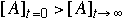?